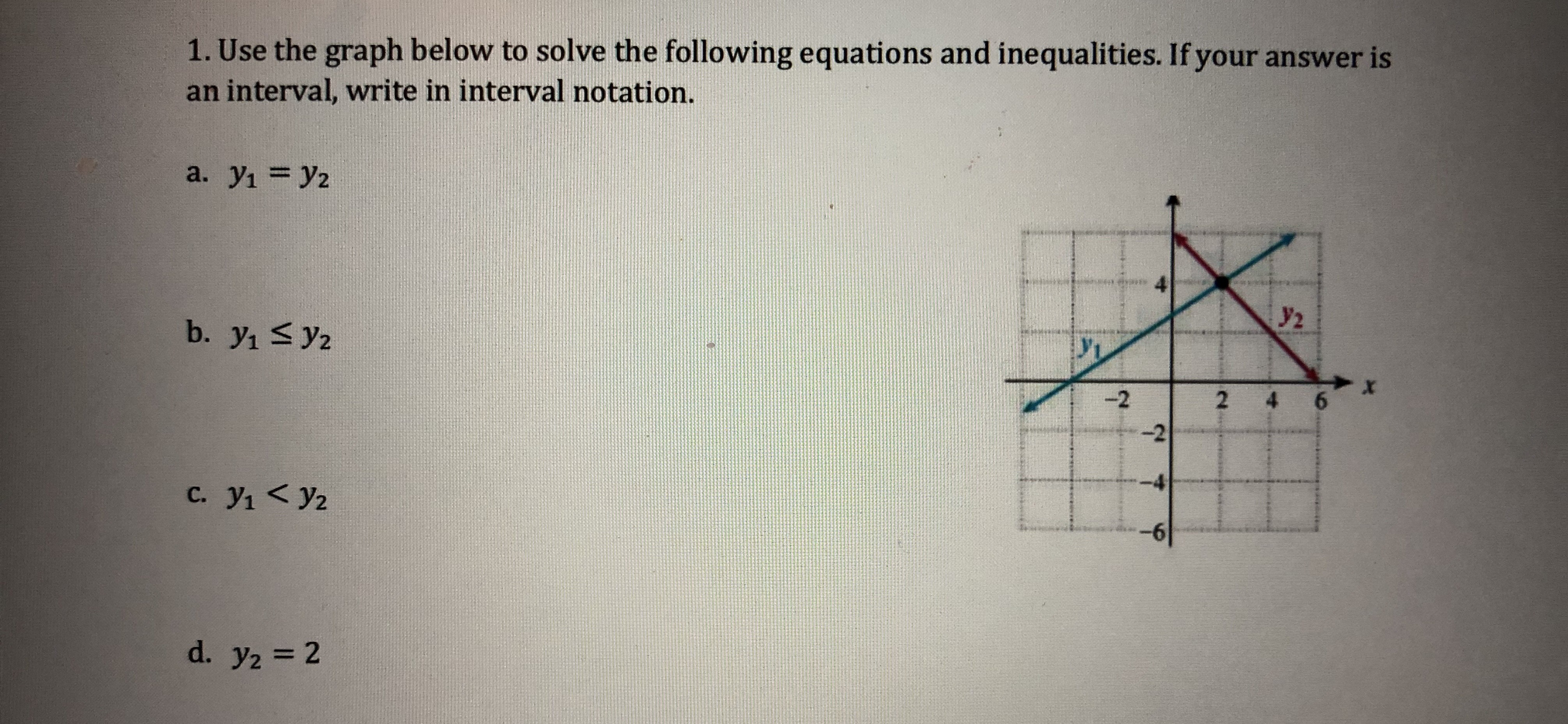1. Use the graph below to solve the following equations and inequalities. If your answer isan interval, write in interval notation.y22-2-4-6C. yi y2

Questionhelp_outlineImage Transcriptionclose1. Use the graph below to solve the following equations and inequalities. If your answer is an interval, write in interval notation. y2 2 -2 -4 -6 C. yi y2 fullscreen
Step 1

To interpret the given graphs algebraically, to solve for the given equations and inequalities

Step 2

The required solutions can be read off directly from the graph: (no need to find the equations of the straight lines y(1) and y(2)

Step 3

(a) y(1)=y(2) corresponds to the point o...

Want to see the full answer?

See Solution

Want to see this answer and more?

Our solutions are written by experts, many with advanced degrees, and available 24/7

See Solution
Tagged in

Equations and In-equations